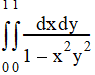# NMath User's Guide

34.2 Integrating Functions of Two Variables (.NET, C#, CSharp, VB, Visual Basic, F#)

The Integrate() method on integrates a given two-variable function over a given region. For example, to compute the double integral:First write the function:

Code Example – C# integration

```private double F( DoubleVector v )
```
```{
```
```  return 1.0 / ( 1.0 - ( v * v * v * v ) );
```
```}
```

Code Example – VB integration

```Function F(V As DoubleVector) As Double
```
```  Return 1.0 / (1.0 - (V(0) * V(0) * V(1) * V(1)))
```
```End Function
```

Then encapsulate the function as a MultiVariableFunction:

Code Example – C# integration

```var function = new MultiVariableFunction(
```
```  new Func<DoubleVector, double>( F ) );
```

Code Example – VB integration

```Dim MultiFunction As New MultiVariableFunction(
```
```  New Func(Of DoubleVector, Double)(F))
```

Finally, compute the integral:

Code Example – C# integration

```var integrator = new TwoVariableIntegrator();
```
```double xLower = 0;
```
```double xUpper = 1;
```
```double yLower = 0;
```
```double yUpper = 1;
```
```double integral = integrator.Integrate( function, xLower, xUpper,
```
```  yLower, yUpper );
```

Code Example – VB integration

```Dim Integrator As New TwoVariableIntegrator()
```
```Dim XLower As Double = 0
```
```Dim XUpper As Double = 1
```
```Dim YLower As Double = 0
```
```Dim YUpper As Double = 1
```
```Dim Integral As Double = integrator.Integrate(MultiFunction,
```
```  XLower, XUpper, YLower, YUpper)
```

The code above explicitly sets the x and y bounds. You can also set the y lower bound, y upper bound, or both, as a function of x. For example, to compute this double integral:First define the function:

Code Example – C# integration

```private double F( DoubleVector v )
```
```{
```
```  return ( 9.0 * v * v ) - ( 3.0 * v );
```
```}
```

Code Example – VB integration

```Function F(V As DoubleVector) As Double
```
```  Return (9.0 * V(0) * V(0)) - (3.0 * V(1))
```
```End Function
```

Then encapsulate the function as a MultiVariableFunction:

Code Example – C# integration

```var function = new MultiVariableFunction(
```
```  new Func<DoubleVector, double>( F ) );
```

Code Example – VB integration

```Dim function As New MultiVariableFunction(
```
```  New Func(Of DoubleVector, Double)(F))
```

Then define the y bounding functions and encapsulate them as OneVariableFunction objects:

Code Example – C# integration

```private double YUpperF( double x )
```
```{
```
```  return Math.Sqrt( 9.0 - ( x * x ) );
```
```}

```

```private double YLowerF( double x )
```
```{
```
```  return -YUpperF( x );
```
```}

```

```var yLowerFunction = new OneVariableFunction(
```
```  new NMathFunctions.DoubleUnaryFunction( YLowerF ) );
```
```var yUpperFunction = new OneVariableFunction(
```
```  new NMathFunctions.DoubleUnaryFunction( YUpperF ) );
```

Code Example – VB integration

```Function YUpperF(X As Double) As Double
```
```  Return Math.Sqrt(9.0 - (X * X))
```
```End Function

```

```Function YLowerF(X As Double) As Double
```
```  Return -YUpperF(X)
```
```End Function

```

```Dim YLowerFunction As New OneVariableFunction(
```
```  New Func(Of Double, Double)(AddressOf YLowerF))
```
```Dim YUpperFunction As New OneVariableFunction(
```
```  New Func(Of Double, Double)(AddressOf YUpperF))
```

Finally, compute the integral:

Code Example – C# integration

```var integrator = new TwoVariableIntegrator();
```
```double xLower = -3;
```
```double xUpper = 3;
```
```double integral = integrator.Integrate( function, xLower, xUpper,
```
```  yLowerFunction, yUpperFunction );
```

Code Example – VB integration

```Dim Integrator As New TwoVariableIntegrator()
```
```Dim XLower As Double = -3
```
```Dim Xupper As Double = 3
```
```Dim Integral As Double = integrator.Integrate(MultiFunction,
```
```  XLower, XUpper, YLowerFunction, YUpperFunction)

```

Top

Top